Chemical Reactions Introduction to Chemical Reactions Chemical Reaction

• Slides: 17Chemical Reactions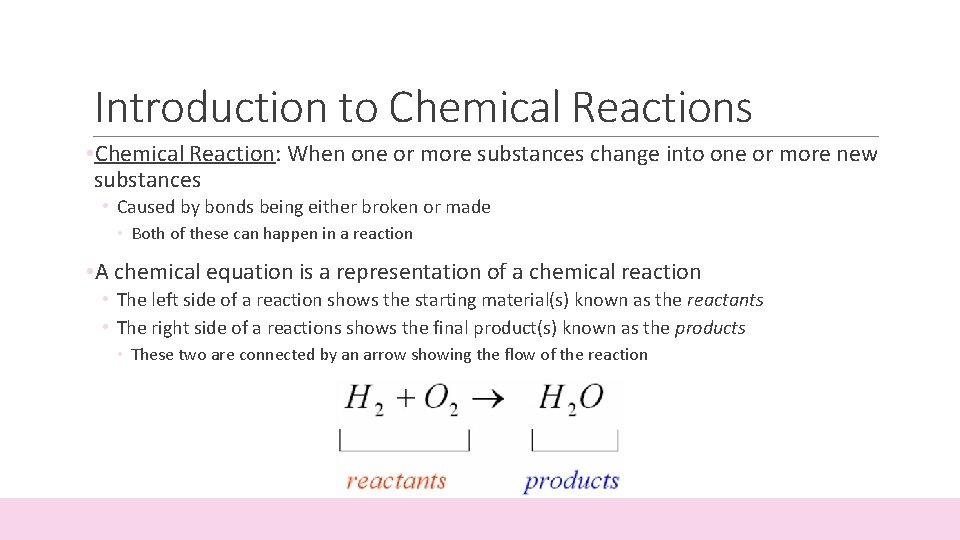Introduction to Chemical Reactions • Chemical Reaction: When one or more substances change into one or more new substances • Caused by bonds being either broken or made • Both of these can happen in a reaction • A chemical equation is a representation of a chemical reaction • The left side of a reaction shows the starting material(s) known as the reactants • The right side of a reactions shows the final product(s) known as the products • These two are connected by an arrow showing the flow of the reaction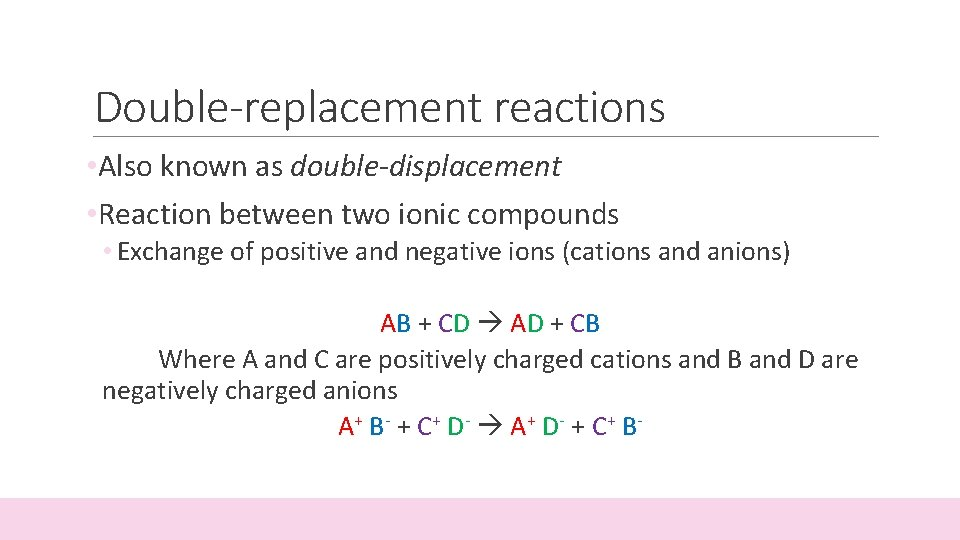Double-replacement reactions • Also known as double-displacement • Reaction between two ionic compounds • Exchange of positive and negative ions (cations and anions) AB + CD AD + CB Where A and C are positively charged cations and B and D are negatively charged anions A + B - + C+ D- A + D- + C+ B -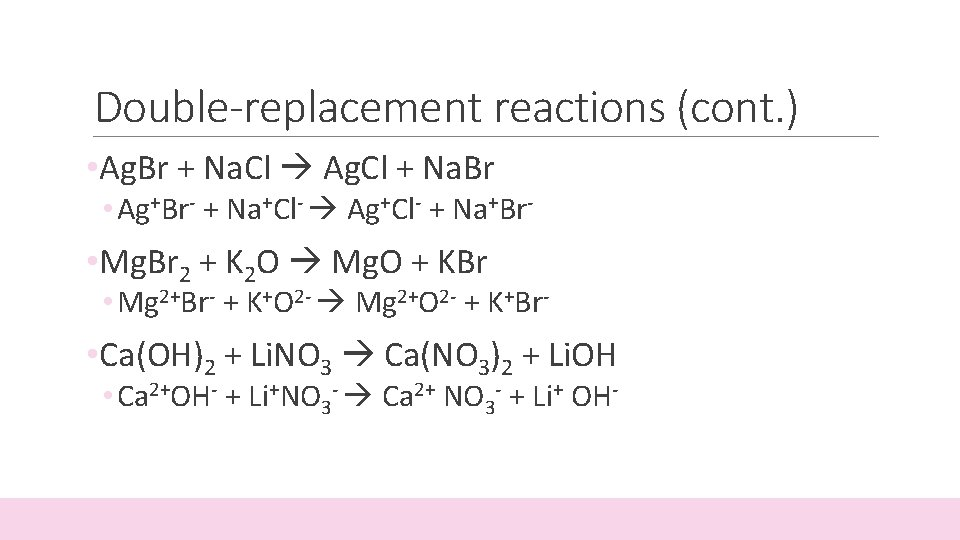Double-replacement reactions (cont. ) • Ag. Br + Na. Cl Ag. Cl + Na. Br • Ag+Br- + Na+Cl- Ag+Cl- + Na+Br- • Mg. Br 2 + K 2 O Mg. O + KBr • Mg 2+Br- + K+O 2 - Mg 2+O 2 - + K+Br- • Ca(OH)2 + Li. NO 3 Ca(NO 3)2 + Li. OH • Ca 2+OH- + Li+NO 3 - Ca 2+ NO 3 - + Li+ OH-Double-replacement reactions (ex. ) • Mg. Cl 2 + KNO 3 • Sr(OH)2 + NH 4 Br • Al. PO 4 + Ca(NO 2)2 • Al. Cl 3 + Na 2 SO 4 • K 2 S + Cs. Br • Fe. O + Cu 3 N 2 • _____ + _____ Cr 4 C 7 + Ag. ClSingle-replacement reactions • Also known as Single-displacement • Chemical change in which one element replaces a second element in a compound A +BC AC + B Examples: ◦ Zn + Cu(NO 3)2 Cu + Zn (NO 3)2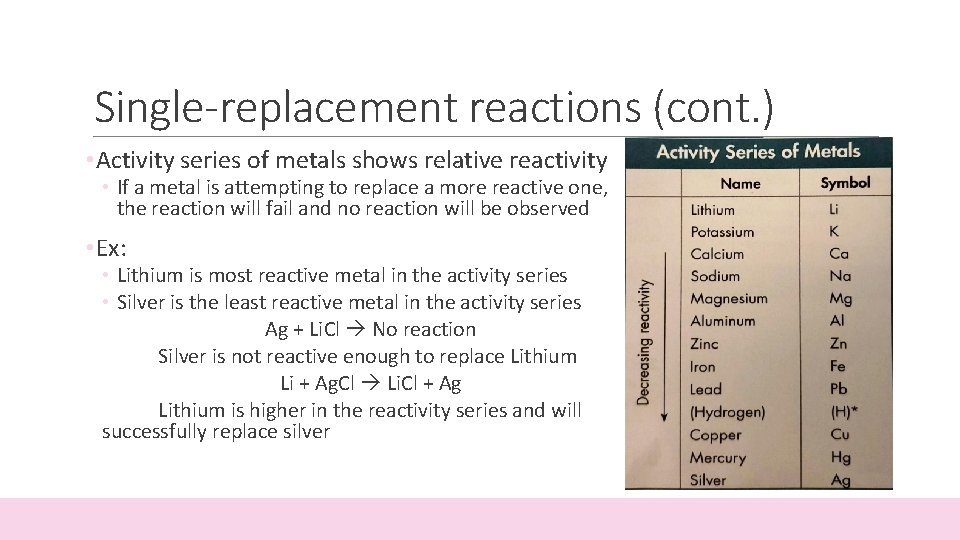Single-replacement reactions (cont. ) • Activity series of metals shows relative reactivity • If a metal is attempting to replace a more reactive one, the reaction will fail and no reaction will be observed • Ex: • Lithium is most reactive metal in the activity series • Silver is the least reactive metal in the activity series Ag + Li. Cl No reaction Silver is not reactive enough to replace Lithium Li + Ag. Cl Li. Cl + Ag Lithium is higher in the reactivity series and will successfully replace silverSingle-replacement reactions (ex. ) • Mg + Ag. Cl • Na + Pb. O • Al + Li 2 S • K 2 O + Li • Hg + Ag 2 Se • Hg will become Mercury (II)/ Mercuric • Zn + Ca. O • Al + Cu. Br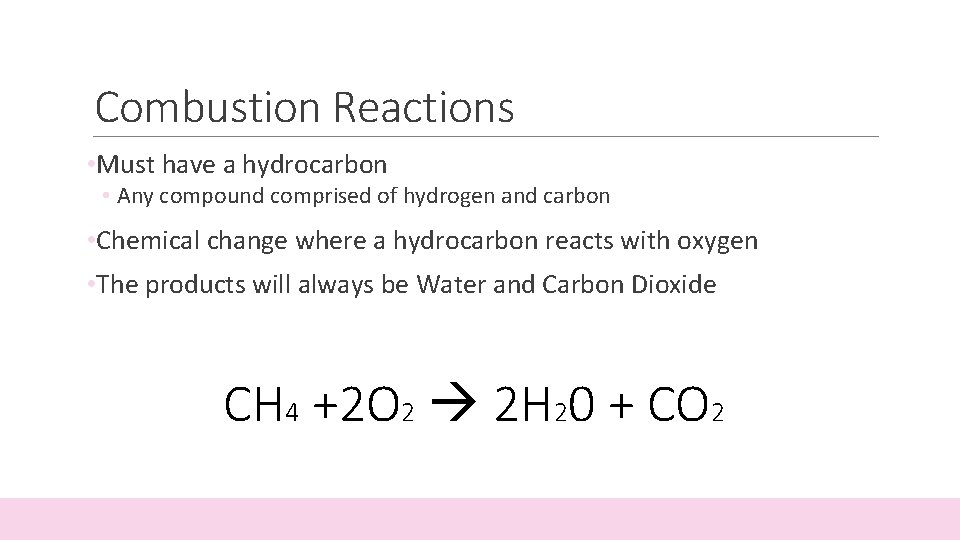Combustion Reactions • Must have a hydrocarbon • Any compound comprised of hydrogen and carbon • Chemical change where a hydrocarbon reacts with oxygen • The products will always be Water and Carbon Dioxide CH 4 +2 O 2 2 H 20 + CO 2Combustion Reactions (cont. ) • How to balance combustion reactions 1. Balance number of carbons 2. Balance number of hydrogens 3. Balance number of oxygens 4. If number of oxygens is an odd number, multiply every coefficient by 2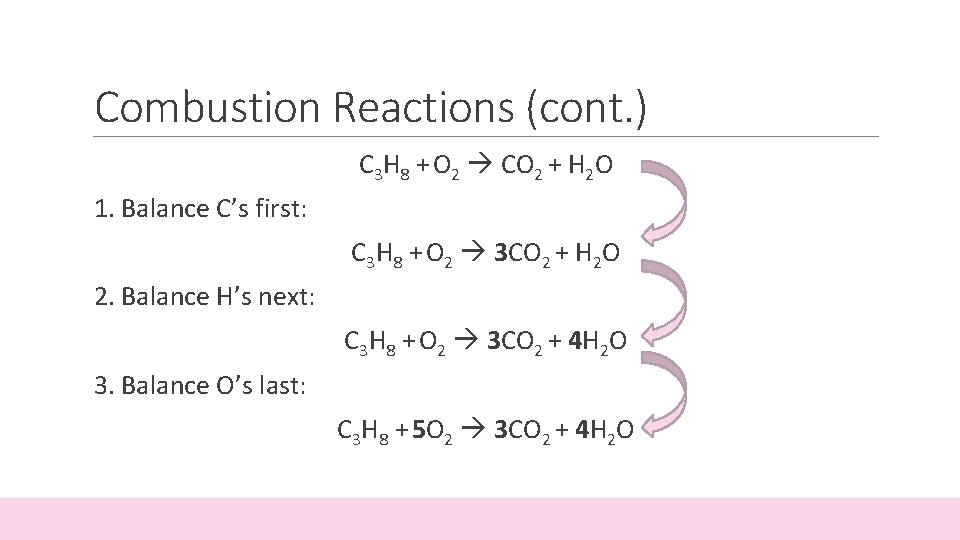Combustion Reactions (cont. ) C 3 H 8 + O 2 CO 2 + H 2 O 1. Balance C’s first: C 3 H 8 + O 2 3 CO 2 + H 2 O 2. Balance H’s next: C 3 H 8 + O 2 3 CO 2 + 4 H 2 O 3. Balance O’s last: C 3 H 8 + 5 O 2 3 CO 2 + 4 H 2 O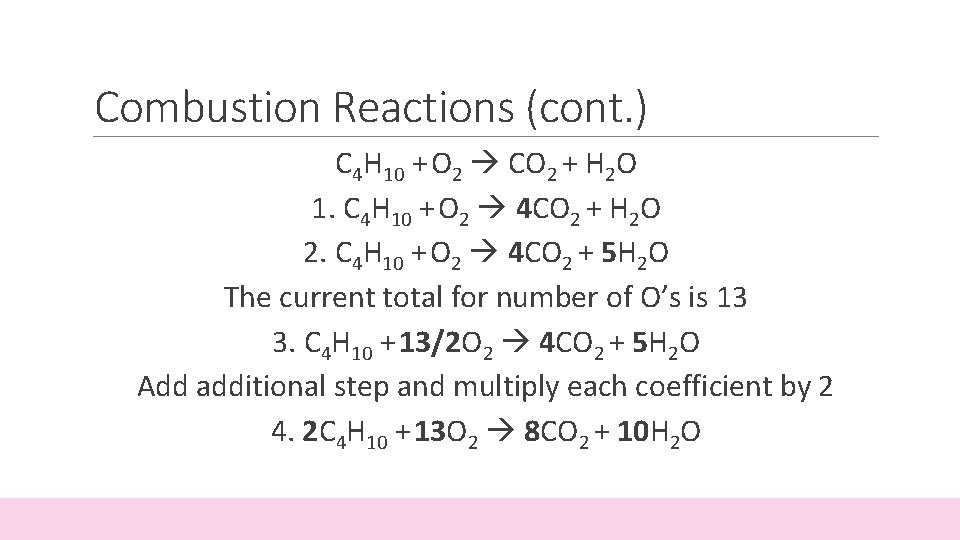Combustion Reactions (cont. ) C 4 H 10 + O 2 CO 2 + H 2 O 1. C 4 H 10 + O 2 4 CO 2 + H 2 O 2. C 4 H 10 + O 2 4 CO 2 + 5 H 2 O The current total for number of O’s is 13 3. C 4 H 10 + 13/2 O 2 4 CO 2 + 5 H 2 O Add additional step and multiply each coefficient by 2 4. 2 C 4 H 10 + 13 O 2 8 CO 2 + 10 H 2 O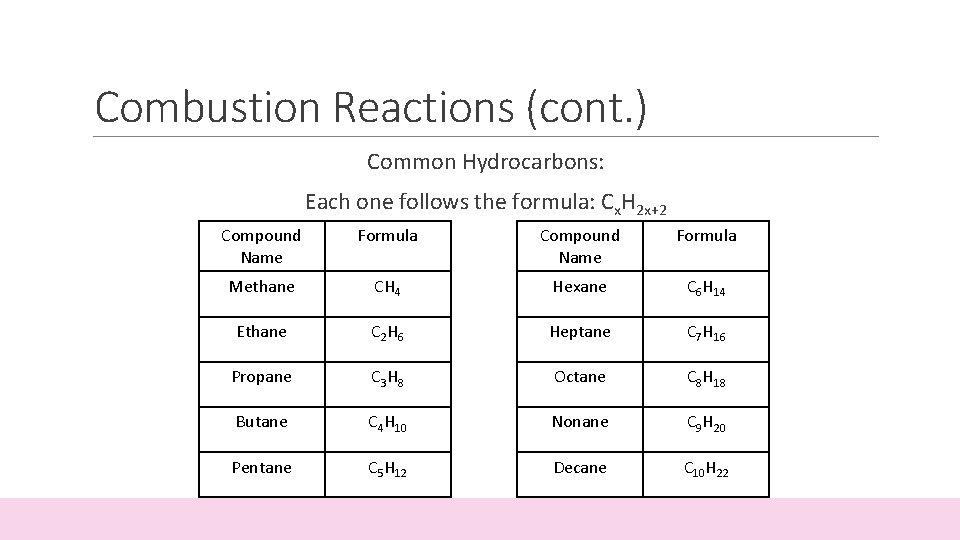Combustion Reactions (cont. ) Common Hydrocarbons: Each one follows the formula: Cx. H 2 x+2 Compound Name Formula Methane CH 4 Hexane C 6 H 14 Ethane C 2 H 6 Heptane C 7 H 16 Propane C 3 H 8 Octane C 8 H 18 Butane C 4 H 10 Nonane C 9 H 20 Pentane C 5 H 12 Decane C 10 H 22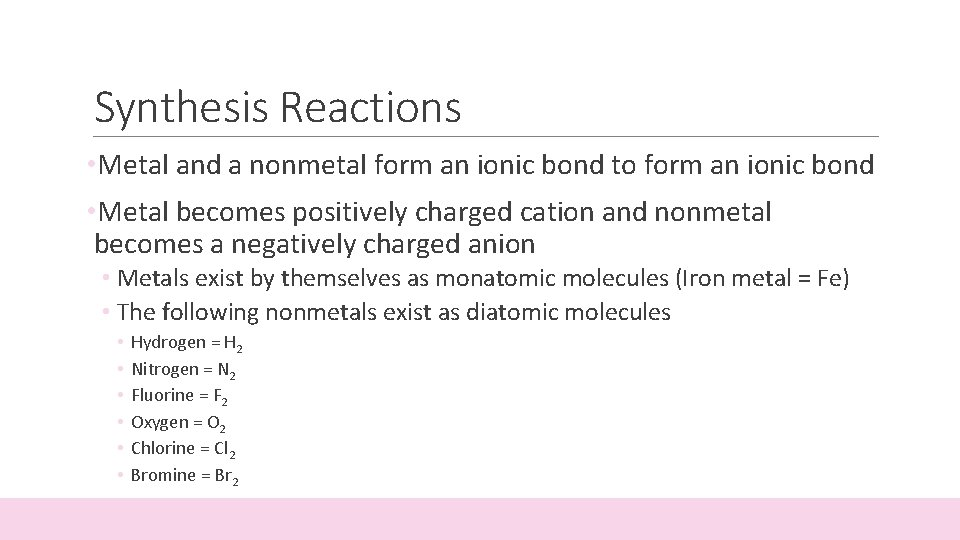Synthesis Reactions • Metal and a nonmetal form an ionic bond to form an ionic bond • Metal becomes positively charged cation and nonmetal becomes a negatively charged anion • Metals exist by themselves as monatomic molecules (Iron metal = Fe) • The following nonmetals exist as diatomic molecules • • • Hydrogen = H 2 Nitrogen = N 2 Fluorine = F 2 Oxygen = O 2 Chlorine = Cl 2 Bromine = Br 2Synthesis Reactions (cont. ) Ex: • 2 Mg + O 2 2 Mg. O • Al + F 2 • Ca + N • Li + O 2 • K + Cl 2 • Fe + O 2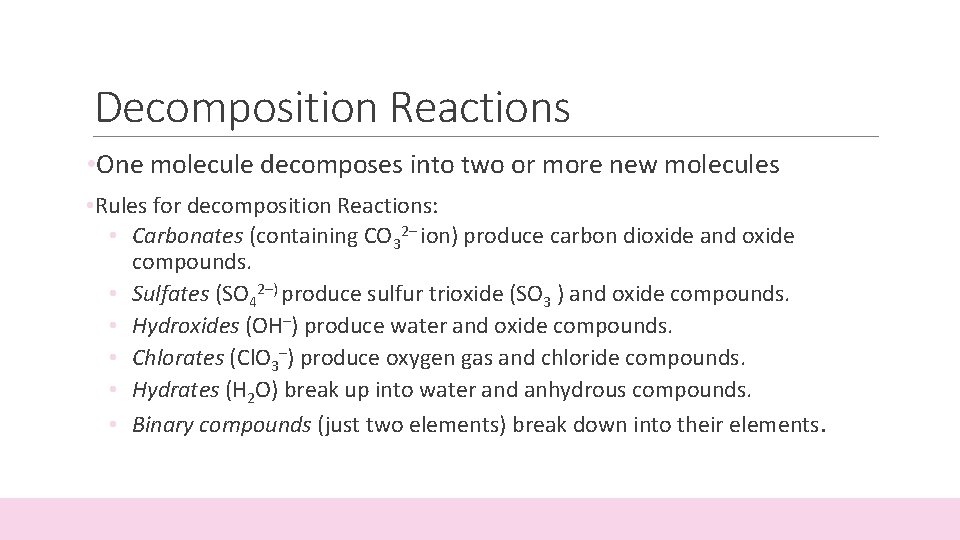Decomposition Reactions • One molecule decomposes into two or more new molecules • Rules for decomposition Reactions: • Carbonates (containing CO 32– ion) produce carbon dioxide and oxide compounds. • Sulfates (SO 42–) produce sulfur trioxide (SO 3 ) and oxide compounds. • Hydroxides (OH–) produce water and oxide compounds. • Chlorates (Cl. O 3–) produce oxygen gas and chloride compounds. • Hydrates (H 2 O) break up into water and anhydrous compounds. • Binary compounds (just two elements) break down into their elements.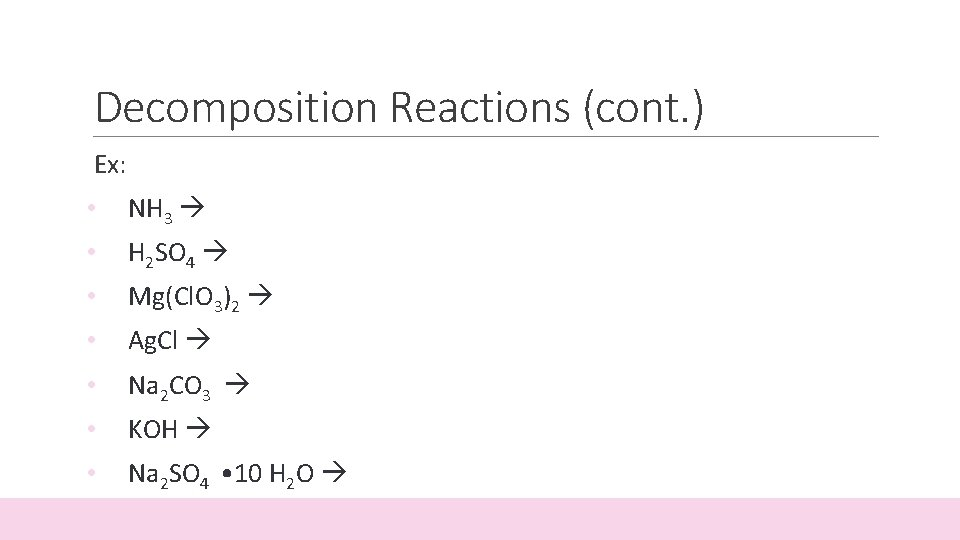Decomposition Reactions (cont. ) Ex: • NH 3 • H 2 SO 4 • Mg(Cl. O 3)2 • Ag. Cl • Na 2 CO 3 • KOH • Na 2 SO 4 • 10 H 2 O